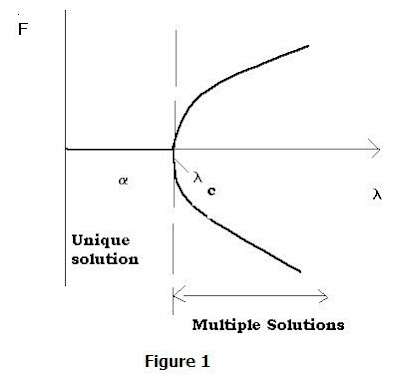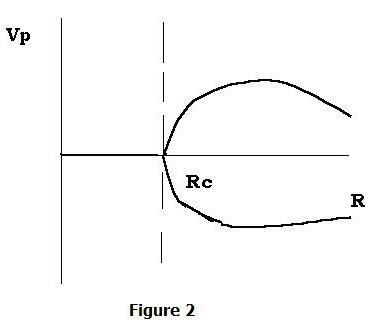## Saturday, February 6, 2010

### Concepts of Order & Disorder II: Order out of ChaosIn the preceding blog I showed examples of simple concepts of order and disorder, and how these can be assessed using a simple system of 8 "spin magnets"- or electrons with differing spins. In this blog, I look at how we can see relative order emerging from chaos, often spontaneously.

The key attribute that distinguishes relatively ordered systems from others is that they tend to occur far from equilibrium- where we defined equilibrium as states at maximum entropy. For example the spin magnet system with 4 spins up and 4 down is at equilibrium. To assist in exposing such systems, one uses what we call "bifurcation diagrams" which shows how the far from equilibrium states diverge in a given system.

A typical form or template for such a diagram is depicted in Fig. 1, where F is some state function, and "lambda" is called the control parameter. Note that the equilibrium solution associated with the above is alpha, valid up to lambda = lambda_c or some critical value of the control parameter. Beyond this value there are multiple solutions, viz. the two separated or bifurcated ones.

An actual example from fluid dynamics is the famous ‘pitchfork bifurcation’, which has nothing to do with demons! It arises by considering the complex interactions of a controlled water channel whose flow continuously recycles. At some point, beyond a critical value of the Reynolds number (R_c), the single flow relinquishes its symmetry and two stable flows result. This is depicted in Fig. 2.

The critical value turns out to be 40.5. As before(earlier blog - Part I), with the Ising model, we see that hidden complexities manifest in a kind of order or self-organization for each of the bifurcation paths. Indeed, each one of these paths can be thought of as mirror images of the same Markov process, tending to some new ‘equilibrium’ displaced from the original one.

An interesting, slightly more complex example from plasma physics is the ‘two stream instability’. In this case the plasma dispersion function F(omega) leads to two bifurcation ‘paths’, including a split symmetrical one and a symmetrical one similar to that shown (Fig. 3)- but in a different direction relative to coordinate axes.

Some investigations- say using computer simulations, disclose interesting properties associated with what we term ‘strange attractors’. For example, two merging regions of plasma that can interact over a large distance to form one single magnetic configuration. Leaving out all the details of the computational plasma physics, a strange attractor for such a budding, complex sunspot field might appear as depicted in Fig. 4(a), with the line axes representing spatial (x, y, z) coordinates.

A sketch of the merging fields, as they might appear confined to two dimensions, including field lines and directions as well as the separatrix, is shown in 4(b). The point is that orderly magnetic fields arise from originally chaotic plasma fields.

The separatrix is analogous to an equilibrium position or barrier between the two contrasting fields. The region with counterclockwise oriented field lines on the left might be called ‘basin of attraction 1’ and the counterpart on the right ‘basin of attraction 2’. At the position where the competing fields are precisely neutralized lies the ‘neutral point’.

These proto-ordered fields are the first signs we note (associated with so-called plages or plage regions) that sunspots may be in the process of forming.

Next: More examples of spontaneous order